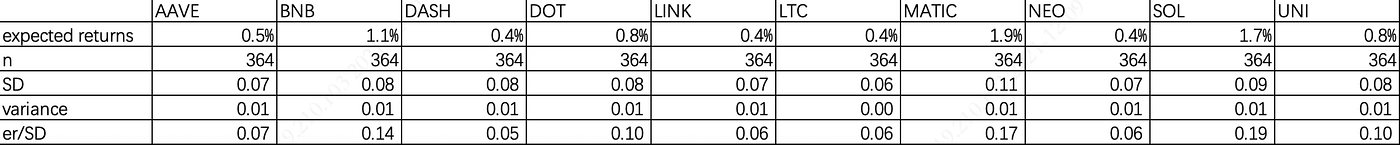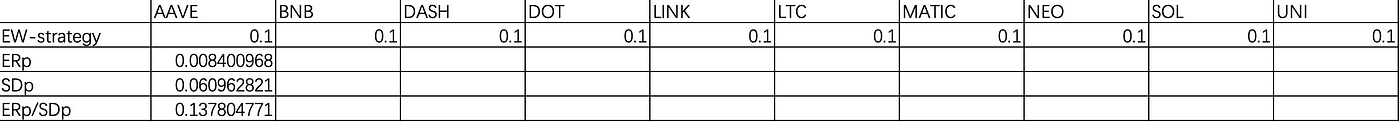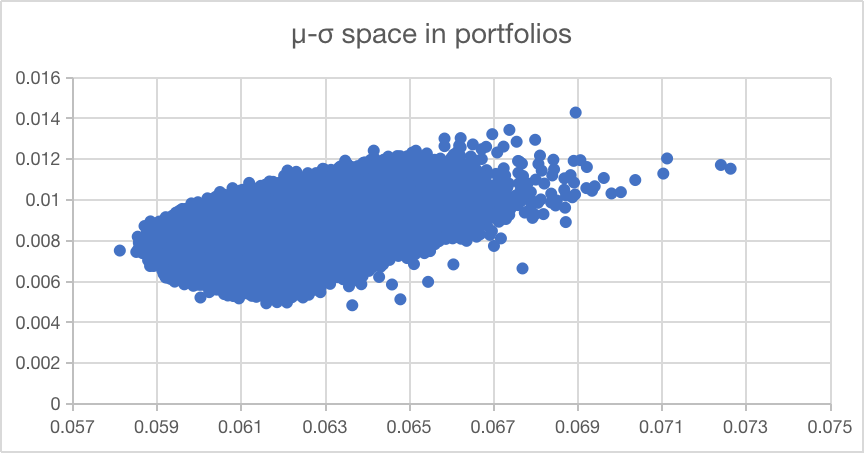# The Efficient Frontier Theory in Crypto Investment

In 1952, Harry Markowitz set a milestone in financial theory. By introducing mean-variance optimization(MVO), often referred to as “Markowitz optimization”, he originated modern portfolio theory(MPT). Even more than 60 years later, his model still widely used among investors, despite its shortcomings. Nowadays, cryptos like bitcoin and ethereum are widely invested all around the world, so how can his theory use in investing cryptos?

This article presents the theory of Markowitz and we will use his theory to present how to optimize the portfolios to get the best return at a lower risk.

A review of Markowitz’s theory

Considering the situation that you have \$100000 to invest to the cryptos. But there are thousands of cryptos all over the world. What should you pick and how much should you invest in a certain kind of crypto? You need a systematic theory to help you to invest.

Naturally, you have two dimensions to pay attention to, which are return and risk. We are informed by others for one thousand times that risk raises with the return raises. Buy what is the exact relationship between risk and return? And how to measure that? Markowitz gave the answer in his article: which are expected return(μ) and its volatility(σ)

Let’s assume that there are only two cryptos to invest: bitcoin and ethereum. From the historical data, we can easily find the expected return and volatility of them. Then we can draw a figure of μ and σ with the individual assets invested.

Then we take portfolios into consideration. In a two-asset portfolio, if the expected return is μ1 and μ2, and we invest three asset with the percentage of w1 and w2, we can easily find that the expected return of portfolio is:

μp = w1 * μ1 + w2 * μ2

with the σ1,σ2 of the single asset, the volatility of the three assets is

vp = w1*w1*σ1* σ1 + w2*w2*σ2*σ2+ 2*w1*w2*Cov(1,2)

then me draw these portfolios with different weight and draw them in the μ-σ space and we can get the figure like this.

Markowitz looked into this figure and he found the efficient portfolios here. A portfolio is efficient if there is no other portfolio has the same expected return with a lower volatility. Graphically the list of portfolios can be described as the upper frontier of the shape, which is called efficient frontier. the minimum point of volatility is called MVP by Harry Markowitz.

Taking this theory to the crypto world, assuming we have 10 cryptos to invest, which are BNB,NEO,DASH,DOT,LINK,AAVE,LTC,MATIC,UNI and SOL.

Historical data of these assets is at https://www.cryptodatadownload.com/. We only use the data in past one year. Then, we get the daily return to calculate the expected return in one year.expected returns, standard deviation, variance and er/SD of individual cryptos

We then calculate the covariance matrix and correlation matrix. It’s important to calculate the volatility of portfolios during next steps.

First of all, Let’s calculate if we use an equal-weighted portfolio which means we buy ten cryptos with the percentage of 10% for each.equal-weighted portfolios and its expected return, standard deviation, and expected return/ standard deviation

We can see that the portfolio’s SD is lower than that of each single asset and the expected return is average return of all assets, Which makes ERp/SDp running at a higher level.

According to Harry Markowitz’s theory, this is not the best portfolio, there may be some portfolio with a lower SDp and a higher ERp. So we mimic the random experiment to change the weight of assets for 100,000 times.μ-σ space and its efficient frontier of cryptos

We find the MVP located in (0.058,0.00075), which indicates that we can have a portfolio of:

(LTC: 31.6%, AAVE:18.6%, SOL: 17.1%, DASH: 8.6%, UNI: 8.6%, BNB: 7.1%, NEO: 3.3%, DOT: 2.2%, LINK: 1.9%, MATIC: 1.1%)

--

--